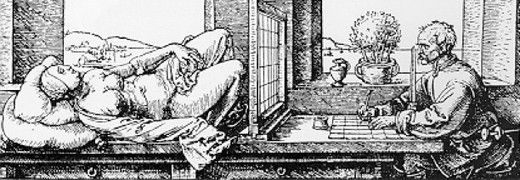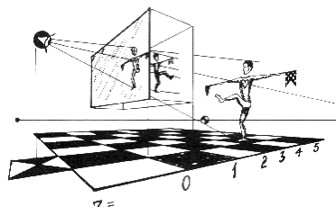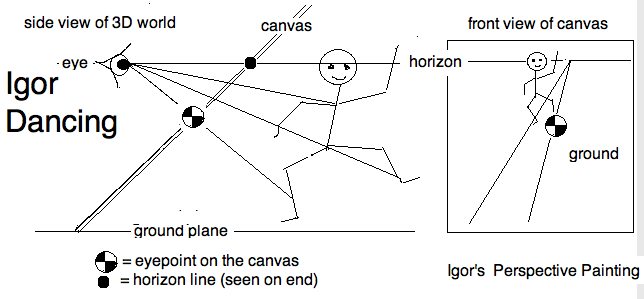This excerpt from "The Topological Picturebook" (Springer Verlag 1987, 2006), was edited by its author, and is included here as the text needed to follow the lessons on perspective drawing that follow.Figure 1: Prairie Horizon A scene in perspective together with a method of marking off equally spaced points on a line which vanishes on the horizon.

## Background

Perspective is the simplest and most direct way of creating the illusion of depth in a picture of spatially extended objects. The more or less correctly placed vanishing points of parallel lines, the estimated regression of evenly spaced points on a line, the elliptically compressed circles, all these tricks of perspective do more than merely please the eye. They help the viewer guess correctly where the artist meant to place things relative to each other. For example, even a modest amount of perspective convergence prevents you from mistaking a three-dimensional picture for a two-dimensional diagram.

Here I am speaking of linear perspective as opposed to aerial perspective. The latter relies more on shading and shadows to give the illusion of depth. Pictures in linear perspective also differ from drawings based on an affine projection, where points in space are cast to the picture plane along mutually parallel lines called the projecting rays, or projectors for short. Since our sun is so far away from earth we can consider its rays to be parallel. Thus shadows on a flat plane are familiar examples of affine projections. Drawings 3D figures in affine projection is particularly easy to do, and often such figure look somewhat realistic. So you have seen them countless number of times in you mathematical textbooks, and your teachers drew such figures on the blackboard (or whiteboard, nowadays.) We shall return to affine projections once you have a grasp of perspective.

In an affine projections there is no convergence: parallel lines in space remain parallel in the picture. Mathematicians often call this a "parallel projection" but the term should be avoided in the company of artists and draftsmen. For them a parallel projection is a perspective projection in which the principal plane of the pictured object is parallel to the picture plane. An affine projection whose projectors are perpendicular to the picture plane is called an orthographic projection, otherwise it is called an oblique projection. This is the generic meaning of "orthographic" although among architects, for example, the term also refers to a composite of two or more orthographic projections at right angles to each other. For the example the plan and elevation of a building.

A discussion of aerial perspective and affine projection is part of the complete exposition in "The Topological Picturebook".

## Linear Perspective

The principle for drawing in perspective is deceptively simple. Painting from life, the artist puts a dot on the canvas where there is a real point to be seen from a fixed position of the eye. Geometrically, this is the point where the line of sight between eye and object crosses the picture plane. Since Albrecht Duerer and the Rennaissance, there has been a steady evolution of ingenious mechanical devices for facilitating this projection.Figure 2: Alberti’s Veil Duerer’s perspective on how to transfer points seen through the veil to points on the canvas.

Today, the photographic camera takes effortless and perfectly perspective pictures of actual scenes: landscapes, buildings, rooms and models. Inexpensive, graphics-oriented microcomputers perform this task for virtual shapes whose points have only mathematical existence.Figure 3: Heart of the Matter A perspective illustration of the principle of linear perspective.

### How a computer draws in perspective

Choose Cartesian coordinates for which the eye, at $(0,0,-\delta)$, is located a focal distance $\delta$ behind the xy-picture plane $z=0$. In computer graphics, the canvas is the vertical monitor screen, and the z-axis is horizontal.

The perspective image, $(X,Y,0)$ , of the point $(x,y,z)$ is scaled in proportion to its distance from the canvas, $Z = \frac{\delta}{\delta + z}$. Thus $(X,Y) = Z(x,y) = (xZ, yZ)$. In this formulation we have compressed the object half space, where $z>0$, into the region where $0 < Z < 1$. An artist might describe this reflection as being "in relief", because the depths of the figures are greatly compressed in the z-direction.

For example, the observer’s eye in Figure 3, at $z=-1$ sees the projected image, on the canvas $z=0$, of a dancer at $z=3$, whose reflected relief (in the box) is sandwiched between the picture plane and its parallel at $z=1$. The transformation from space to reflected relief is invertible, because we can solve the equations for the space coordinates

 $X = \frac{x\delta}{\delta + z}$ $x = \frac{X}{Z}$ $Y = \frac{y\delta}{\delta + z}$ $y = \frac{Y}{Z}$ $Z = \frac{\delta}{\delta + z}$ $z = \delta \frac{1-Z}{Z}$

It could be argued that the recognition of a picture in perspective involves a mental inference about the perspective scaling parameter $Z = \frac{\delta}{\delta + z$.

### Drawing in perspective by hand

Before photography, and especially before computer graphics, the designer had no choice but to use the classical methods of drawing with ruler and compass. The discipline for this, descriptive geometry, was a required subject in every engineering, architecture and industrial design curriculum.

Even so, considering the tedium of building physical models to be photographed, the steep learning curve in using computer tools to design irregularly curved surfaces, or of entering the millions of data points needed by the computer to do it on its own, there are still many instances when drawing by hand is still the better choice. For convenience and speed, the perspective sketch is the best choice also for descriptive topologists.

Instruction for drawing in perspective can be found in primers for students of architecture, the fine arts, and industrial design (in order of increasing utility). The architect rearranges familiar objects in a familiar universe; the artist mostly draws from life. Only the designer faces problems similar to those of the topologist: to (re)create the object in the act of drawing it. Industrial and topological designers both strive to transmit visual information with minimal ambiguity and maximal economy of style. The difference comes in the nature of the pictured objects themselves. A teakettle or an automobile is, after all, part of the viewer’s everyday experience. A picture of a new object of this kind serves chiefly to "reset the parameters" of an idea already in the imagination. Most pictures drawn by the topologist are of unfamiliar objects. Only rarely do they correspond to any physical reality. While a M\"obius band is easily made from a strip of paper, how to sew a disc to its boundary is not a skill taught in the schools.

Hand-drawn perspective plays a special role in descriptive topology. Since perspective cubes, cylinders and cones used for framing a drawing can be copied from the computer screen, it is not absolutely essential. However, it does help the topologist to visualize and then design the object in the first place, by whatever means the finished product will be realized. Finally, I have found that teaching projective geometry, especially to teachers, artists and computer graphicists, is best done in terms of perspective drawing.

Comment

It is impractical here to present the theory of perspective from a historical viewpoint. Instead, we start with Kepler’s concept of ideal points, lines and planes, and the notion of a projective geometry based on this. Readers who have a more extensive knowledge of projective geometry will be reminded, occasionally, of what they already know. Readers familiar only with the earlier introduction to this subject are invited to ignore such references.

### Vanishing points

Recall that projective space consists of ordinary 3-space augmented by an ideal point for each set of mutually parallel lines. An ideal point is joined to a real point by its representative through that point. Two ideal points, represented by two non-parallel real lines, are joined by an ideal line corresponding to the set of mutually parallel planes determined by the two lines. These ideal lines and points reside on an ideal plane, also thought of as the plane-at-infinity. In analogy to the projective plane, whose points correspond to antipodal pairs of points on a sphere under central projection, projective space has a Euclidean model in 4-space, consisting of antipodally identified objects on the 3-sphere.Figure 4: Igor Dancing: A geometric formulation of Alberti’s Veil

That two parallel lines "meet at infinity" and that two parallel planes have a common "infinite line" has the following perspective interpretation. The ray emanating from the observer’s eye, the sight line, passes through the image point on the canvas to the object point in space. As the object point at the end of the sightline moves away from the observer along a straight line in space, its trace on the picture plane moves along a line segment until it stops at the vanishing point for the object line. This happens when the sightline is parallel in space to the object line being drawn in perspective. For this reason, the vanishing point remains fixed as long as the object line moves parallel to itself in space. So, the vanishing point, which is on the canvas, represents the ideal point on the object line.

As long as the object line rotates in an object plane, its vanishing point moves along a line, called the horizon line for the object plane. In this way, the horizon line on the canvas represents the ideal line on the object plane in space. Thus the picture plane comes into 1:1 correspondence with the ideal plane under a projection along lines of sight. The sight line for a point moving along an object line which is parallel to the picture plane becomes parallel to the picture plane. So the vanishing point of an object line parallel to the picture plane must be the ideal point of its picture. Its ideal line is the horizon for real planes parallel to the picture plane. In a perspective drawing, only lines parallel to the canvas remain parallel. Any other pair of objectively parallel lines meet, in perspective, at their common vanishing point. A line parallel to a plane vanishes on the horizon for that plane.

What follows is a sequence of lessons on drawing in perspective which are of independent interest. They also illustrate a number of profound geometrical ideas, which presented in a less pictorial ways soon loose their charm.

### Computer assisted hand drawing

For practical and aesthetic reasons, we enlist the graphics computer to assist drawing in the classical style; equivalent to drawing board, T-square, rulers, pencil (and eraser), and india ink. Even if the classical drawing instruments are used for the final work, the ability of the computer to produce infinitely many different "what-if" versions is already worth the additional effort. We will refer to exercises to be done in KSEG and in iPaint (Paint). Other tools are equally or even more useful, though the generally have a longer learning curve, and a steeper ascent (and price.)